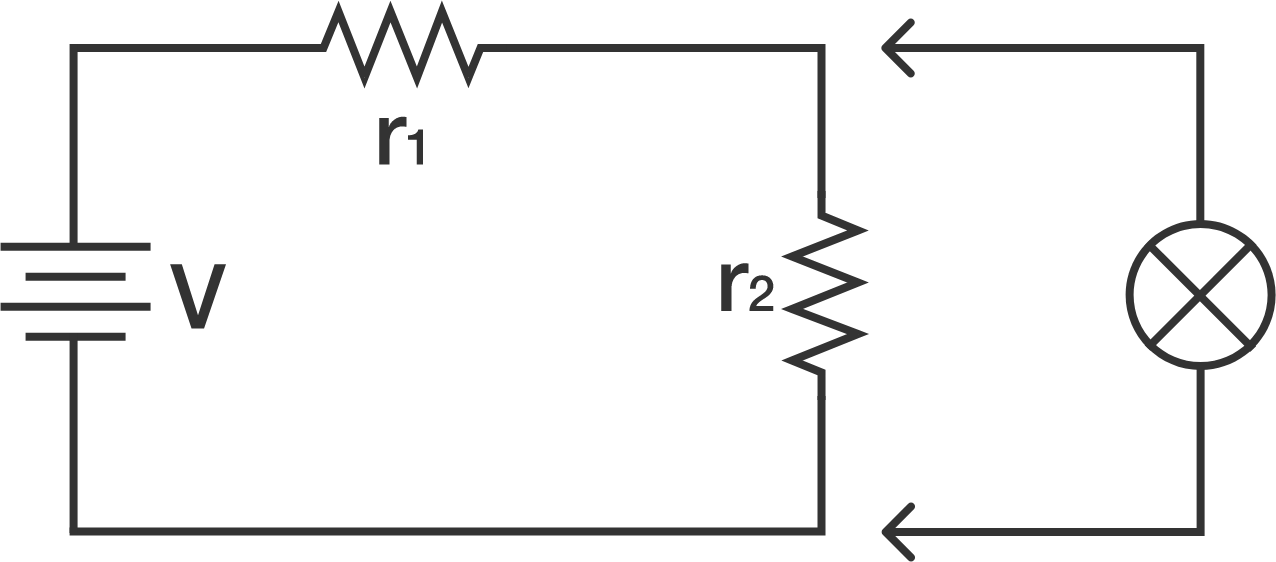# Circuit Solution via Incremental Quantities

In the circuit shown below, $r_1$ and $r_2$ are resistors such that $\frac{r_1}{r_2}=4$. If we connect a lamp as shown, the current through resistor $r_1$ increases by $\SI{0.1}{\ampere}$.

Find the current through the lamp (in amperes).×